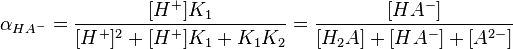## Calculating Equilibrium Concentrations of Polyprotic Acids

#### Learning Objective

• Solve equilibrium problems using the appropriate approximations for weak and strong polyprotic acids.

#### Key Points

• Polyprotic acids contain multiple acidic protons that can sequentially dissociate from the compound with unique acid dissociation constants for each proton.
• Due to the variety of possible ionic species in solution for each acid, precisely calculating the concentrations of different species at equilibrium can be very complicated.
• Certain simplifications can make the calculations easier; these simplifications vary with the specific acid and the solution conditions.

#### Terms

• ionic specieschemical species with a residual charge; in acid-base equilibria, the charge resulting from the loss or addition of electrons from chemical compounds
• equilibriumthe state of a reaction in which the rates of the forward and reverse reactions are the same
• polyprotic acidsan acid with multiple acidic protons

Polyprotic acids can lose more than one proton. The first proton’s dissociation may be denoted as Ka1 and the constants for successive protons’ dissociations as Ka2, etc. Common polyprotic acids include sulfuric acid (H2SO4), and phosphoric acid (H3PO4).

When determining equilibrium concentrations for different ions produced by polyprotic acids, equations can become complex to account for the various components. For a diprotic acid for instance, we can calculate the fractional dissociation (alpha) of the species HA using the following complex equation:Equation for finding the fractional dissociation of HA-The above concentration can be used if pH is known, as well as the two acid dissociation constants for each dissociation step; oftentimes, calculations can be simplified for polyprotic acids, however.

We can simplify the problem, depending on the polyprotic acid. The following examples indicate the mathematics and simplifications for a few polyprotic acids under specific conditions.

## Diprotic Acids With a Strong First Dissociation Step

As we are already aware, sulfuric acid’s first proton is strongly acidic and dissociates completely in solution:

H2SO4 → H+ + HSO4

However, the second dissociation step is only weakly acidic:

$HSO_4^- \rightleftharpoons H^+ + SO_4^{2-}$  Ka2 = 1.20×10-2   pKa2 = 1.92

Because the first dissociation is so strong, we can assume that there is no measurable H2SO4 in the solution, and the only equilibrium calculations that need be performed deal with the second dissociation step only.

## Determining Predominant Species From pH and pKa

Phosphoric acid, H3PO4, has three dissociation steps:

$H_3PO_4 \rightleftharpoons H^+ + H_2PO_4^-$  pKa1 = 2.12

$H_2PO_4^- \rightleftharpoons H^+ + HPO_4^{2-}$   pKa2 = 7.21

$HPO_4^{2-} \rightleftharpoons H^+ + PO_4^{3-}$  pKa3 = 12.67

Thus, in an aqueous solution of phosphoric acid, there will theoretically be seven ionic and molecular species present: H3PO4, H2PO4, HPO42-, PO43-, H2O, H+, and OH.

At a pH equal to the pKa for a particular dissociation, the two forms of the dissociating species are present in equal concentrations, due to the following mathematical observation. Take for instance the second dissociation step of phosphoric acid, which has a pKa2 of 7.21:

$pK_{a2}=-log\left(\frac{[H^+][HPO_4^{2-}]}{[H_2PO_4^-]}\right)=7.21$

$pH=-log[H^+]=7.21$

By the property of logarithms, we get the following:

$pH-pK_{a2}=-log\left(\frac{[HPO_4^{2-}]}{[H_2PO_4^-]}\right)=0$

$\frac{[HPO_4^{2-}]}{[H_2PO_4^-]}=1$

Thus, when pH = pKa2, we have the ratio [HPO42-]/[H2PO4] = 1.00; in a near-neutral solution, H2PO4 and HPO42- are present in equal concentrations. Very little undissociated H3PO4 or dissociated PO43- will be found, as is determined through similar equations with their given Ka‘s.

The only phosphate species that we need to consider near pH = 7 are H2PO4 and HPO42-. Similarly, in strongly acidic solutions near pH = 3, the only species we need to consider are H3PO4 and H2PO4. As long as the pKa values of successive dissociations are separated by three or four units (as they almost always are), matters are simplified. We need only consider the equilibrium between the two predominant acid/base species, as determined by the pH of the solution.Phosphoric acidThe chemical structure of phosphoric acid indicates it has three acidic protons.

## Diprotic Acids With a Very Weak Second Dissociation Step

When a weak diprotic acid such as carbonic acid, H2CO3, dissociates, most of the protons present come from the first dissociation step:

$H_2CO_3 \rightleftharpoons H^+ + HCO_3^-$  pKa1 = 6.37

Since the second dissociation constant is smaller by four orders of magnitude (pKa2 = 10.25 is larger by four units), the contribution of hydrogen ions from the second dissociation will be only one ten-thousandth as large. Consequently, the second dissociation has a negligible effect on the total concentration of H+ in solution, and can be ignored.